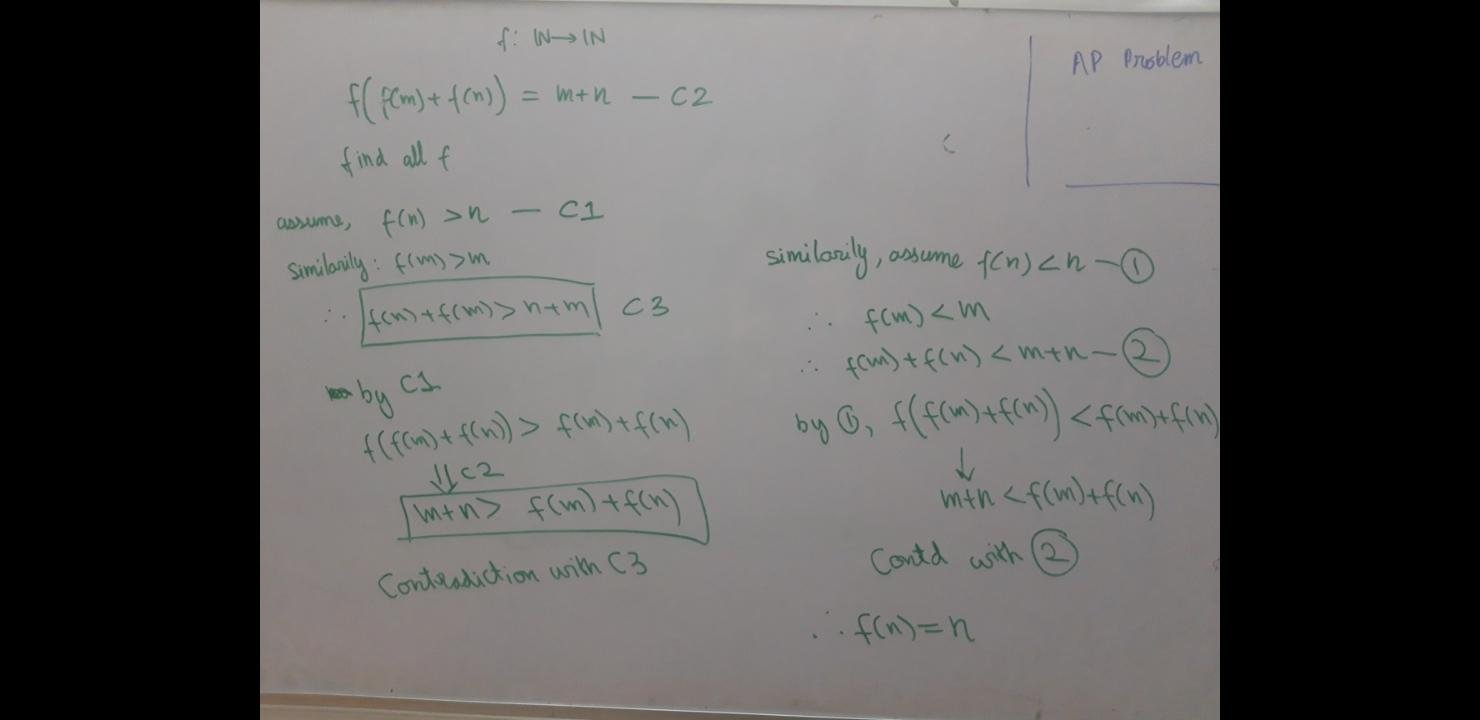# Functional equations are weird

Home Forums Math Olympiad, I.S.I., C.M.I. Entrance Number Theory Functional equations are weird

Viewing 3 posts - 1 through 3 (of 3 total)
• Author
Posts
• #29158

Is the following method correct?I mean is it enough to imply that f(n)=N

Do we have to prove wether f is ever increasing or ever decreasing before doing this. Like could it be that f is sometimes greater than n and sometimes less, and we proved that is not greater when it is less.

Kind of like sin(x). It increases and decreases. Do we have to consider the that f might also be increasing and decreasing above and below n.

or is it correct and that is taken care of when we take the case f(n)>n.

Help would really be appreciated.

Thanks.

#29183

No, just checking for the case where f is decreasing or increasing is not sufficient. As you pointed out, we need to consider the case (if possible) where f is just some random arrangement and doesn’t have monotonic property.

Hints

• If  ‘f’  a one-one function? Observe that if f(m)=f(n) implies m=n then, the given  function is one-one.
• Observe that  $$f[ f(m+k)+f(n-k) ]=m+n=f[ f(m)+f(n) ]$$. What can we conclude?

Using further manipulations you can finally show that f(n)=n. The complete solution has been attached below

###### Attachments:
You must be logged in to view attached files.
#29266
Viewing 3 posts - 1 through 3 (of 3 total)
• You must be logged in to reply to this topic.